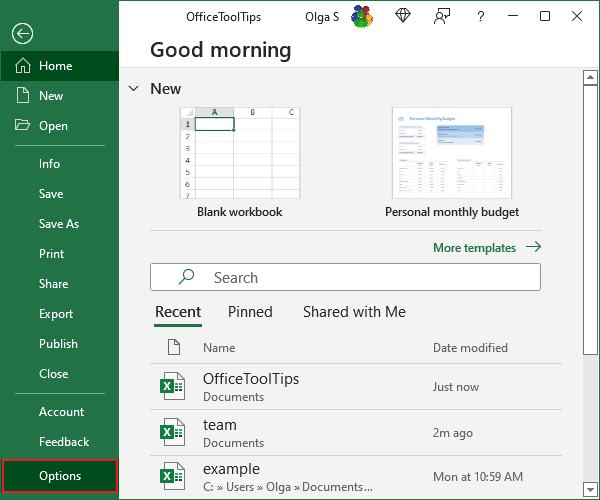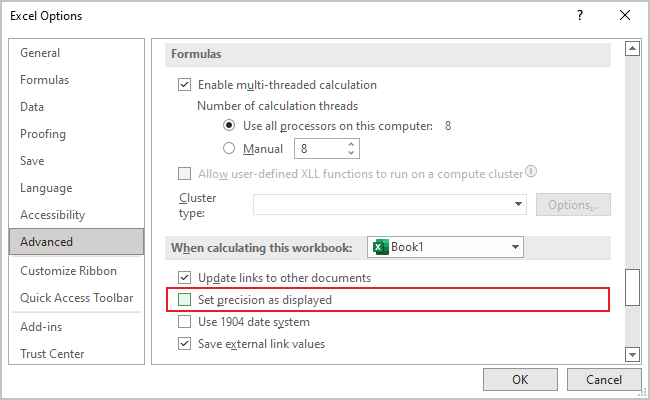# How to avoid misleading numbers

Excel
Applying a number format to a cell doesn't change the value but only how the value appears in the worksheet. Formatting can play a joke with you, e.g., the sum of values seems incorrect because Excel displays a limited number of decimal places, and their sum is not equal to the real sum.

For example, if a cell contains .874543, you might format it to appear as 87%. If that cell is used in a formula, the formula uses the full value (.874543), not the displayed value (.87).

In some situations, formatting may cause Excel to display calculation results that appear incorrect, such as when totaling numbers with decimal places. For example, if values are formatted to display two decimal places, you may not see the actual numbers used in calculations. But because Excel uses the full precision of the values in its formula, the sum of two values may appear incorrect.

Several solutions to this problem are available:

• You can format the cells to display more decimal places.
• You can use the ROUND function on individual numbers and specify the number of decimal places Excel should round to.
• Or you can instruct Excel to change the worksheet values to match their displayed format.

To do this, do the following:

1.   On the File tab, click the Options button:2.   On the Advanced tab, in the When calculating this workbook group, check the Set precision as displayed checkbox: